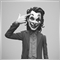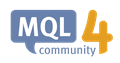# How To Use iTime in The Future532

I want to start the calculation of ShiftTime from spesific datetime in the future for BeginTime and skip calculation in current time before BeginTime (return nothing).

datetime BeginTime = D'2018.09.01 00:00';
datetime ShiftTime = iTime(NULL, PERIOD_MN1, 1);

I try this code, is it correct?

int ShiftBar=iBarShift(NULL, PERIOD_MN1, BeginTime);
datetime ShiftTime = iTime(NULL, PERIOD_MN1, ShiftBar+1);16429

I want to start the calculation of ShiftTime from spesific datetime in the future for BeginTime and skip calculation in current time before BeginTime (return nothing).

datetime BeginTime = D'2018.09.01 00:00';
datetime ShiftTime = iTime(NULL, PERIOD_MN1, 1);

I try this code, is it correct?

int ShiftBar=iBarShift(NULL, PERIOD_MN1, BeginTime);
datetime ShiftTime = iTime(NULL, PERIOD_MN1, ShiftBar+1);532

Thanks for the answer but I am not got your point. What's the meaning of red & yellow highlight?

I want to ignore previous 1 month until  specified date (BeginTime), in this case is 1 September 2018 and start this calculation from specified date (BeginTime) and then calculate previous 1 month from that date continuously into future.

Here's the full code:

```datetime BeginTime = D'2018.09.01 00:00';
datetime ShiftTime = iTime(NULL, PERIOD_MN1, 1);

int ShiftBar=iBarShift(NULL, PERIOD_MN1, BeginTime);
datetime EndTime = iTime(NULL, PERIOD_MN1, ShiftBar+1);

for(int i=0; i<OrdersHistoryTotal(); i++)
{
if (OrderSelect(i, SELECT_BY_POS, MODE_HISTORY))
{
if(OrderOpenTime() >= EndTime)
{
totalLots += OrderLots();
}
}
}

if (totalLots<1.0)
{
Alert("Total Lot less than 1.0 lot");
return(1);
}
```16429

Thanks for the answer but I am not got your point. What's the meaning of red & yellow highlight?

I want to ignore previous 1 month until  specified date (BeginTime), in this case is 1 September 2018 and start this calculation from specified date (BeginTime) and then calculate previous 1 month from that date continuously into future.

Here's the full code:

1. Your Begin Time is not yet arrive (according to server time).
2. int ShiftBar is exactly 1.
3. datetime EndTime is 2 months back (read timeseries on iTime)iTime - Timeseries and Indicators Access - MQL4 Reference
• docs.mql4.com
Time value for the bar of specified symbol with timeframe and shift. If local history is empty (not loaded), function returns 0. To check errors, one has to call the GetLastError() function.